# JP2012058845A - Image processing device and method, and program - Google Patents

## Info

Publication number
JP2012058845A
JP2012058845A JP2010199106A JP2010199106A JP2012058845A JP 2012058845 A JP2012058845 A JP 2012058845A JP 2010199106 A JP2010199106 A JP 2010199106A JP 2010199106 A JP2010199106 A JP 2010199106A JP 2012058845 A JP2012058845 A JP 2012058845A
Authority
JP
Japan
Prior art keywords
block
pixel
local region
histogram
input image
Prior art date
Legal status (The legal status is an assumption and is not a legal conclusion. Google has not performed a legal analysis and makes no representation as to the accuracy of the status listed.)
Withdrawn
Application number
JP2010199106A
Other languages
Japanese (ja)
Inventor

Original Assignee
Sony Corp
ソニー株式会社
Priority date (The priority date is an assumption and is not a legal conclusion. Google has not performed a legal analysis and makes no representation as to the accuracy of the date listed.)
Filing date
Publication date
Application filed by Sony Corp, ソニー株式会社 filed Critical Sony Corp
Priority to JP2010199106A priority Critical patent/JP2012058845A/en
Publication of JP2012058845A publication Critical patent/JP2012058845A/en
Application status is Withdrawn legal-status Critical

• 238000004364 calculation methods Methods 0 abstract 3
• 230000000875 corresponding Effects 0 abstract 1
• 238000000926 separation method Methods 0 abstract 1

## Images

•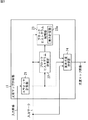•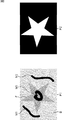••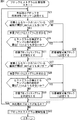•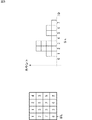•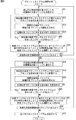•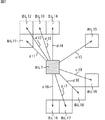•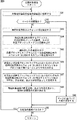•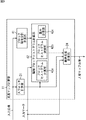••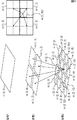•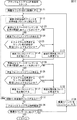•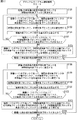•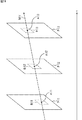•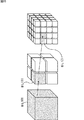•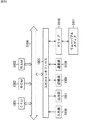## Classifications

• GPHYSICS
• G06COMPUTING; CALCULATING; COUNTING
• G06TIMAGE DATA PROCESSING OR GENERATION, IN GENERAL
• G06T7/00Image analysis
• G06T7/10Segmentation; Edge detection
• G06T7/143Segmentation; Edge detection involving probabilistic approaches, e.g. Markov random field [MRF] modelling
• GPHYSICS
• G06COMPUTING; CALCULATING; COUNTING
• G06TIMAGE DATA PROCESSING OR GENERATION, IN GENERAL
• G06T7/00Image analysis
• G06T7/10Segmentation; Edge detection
• G06T7/11Region-based segmentation
• GPHYSICS
• G06COMPUTING; CALCULATING; COUNTING
• G06TIMAGE DATA PROCESSING OR GENERATION, IN GENERAL
• G06T7/00Image analysis
• G06T7/10Segmentation; Edge detection
• G06T7/194Segmentation; Edge detection involving foreground-background segmentation
• GPHYSICS
• G06COMPUTING; CALCULATING; COUNTING
• G06TIMAGE DATA PROCESSING OR GENERATION, IN GENERAL
• G06T2207/00Indexing scheme for image analysis or image enhancement
• G06T2207/20Special algorithmic details
• G06T2207/20021Dividing image into blocks, subimages or windows
• GPHYSICS
• G06COMPUTING; CALCULATING; COUNTING
• G06TIMAGE DATA PROCESSING OR GENERATION, IN GENERAL
• G06T2207/00Indexing scheme for image analysis or image enhancement
• G06T2207/20Special algorithmic details
• G06T2207/20076Probabilistic image processing

## Abstract

PROBLEM TO BE SOLVED: To enable separation of an object image from an input image with high accuracy.SOLUTION: A swatch calculation part 21 calculates a swatch from an input image as a color swatch column and sets a corresponding swatch ID. A block histogram calculation part 22 divides the input image into blocks, calculates a histogram for each swatch ID of the blocks as a block histogram, and sets an incomputable block as a block to be interpolated. A block histogram interpolation part 23 calculates weights for the block to be interpolated, in proportion to distances from the blocks for which the block histograms are computed, and calculates the block histogram of the block to be interpolated with each of the weights. A pixel likelihood calculation part 24 calculates pixel likelihood for each pixel based on the number of counts for the each swatch ID of the block histograms for the each block. The invention is applicable to an image processing device.

## Description

The present invention relates to an image processing apparatus, method, and program, and more particularly, to an image processing apparatus, method, and program that enable high-precision segmentation.

Separating an object image (subject image or foreground image) in an image from a background image is useful in many applications such as image editing and video processing.

In many methods, the object image is separated from the background image using the color information of the image as one of the cues.

For the color information, a probability model is calculated using the color of the entire image or a specified part of the pixel, and the likelihood obtained from the model is calculated and used in accordance with the color of the pixel. The object color and background color for calculating the probabilistic model are pre-processed by the user marking the image object and some pixels in the background area or by executing a predetermined software program. It is specified.

In a simple image, the subject can be separated from the likelihood difference based on the difference in color distribution between the background image and the object image.

In a more general image, the color distribution is complex, and the likelihood determined from the color distribution of the entire image often provides only an ambiguous separation result of the object image and the background image.

Therefore, a method of improving the color separation performance by obtaining such a color distribution model globally using the colors of the entire image, instead of obtaining it locally for each image region, can be considered.

One way to calculate a local color distribution model is to use small changes in the motion of an object in successive frames of the video, place a group of small windows on the boundary of the object predicted from the motion, and A method has been proposed in which a color distribution model is calculated in a window and an object image and a background image are separated and optimized (see Patent Document 1).

Further, a method has been proposed in which a Gaussian Mixture Model (GMM) is used as a color distribution model to calculate and use a five-dimensional probability model including not only RGB three-dimensional but also XY image space positions (patent). Reference 2).

US7609888, SEPARATING A VIDEO OBJECT FROM A BACKGROUND OF A VIDEO SEQUENCE US2007 / 0237393, IMAGESEGMENTATION USING SPATIAL-COLOR GAUSSIAN MIXTURE MODELS

However, in order to apply the method of Patent Document 1 to a still image, it is necessary to give the general shape of the object and specify the position of the small window at a position corresponding to the object boundary line. Further, since the outline of the object is determined only from within the small window, it is necessary to specify a considerably large window size for safety. Thus, in the method of Patent Document 1, there are many conditions to be input.

Further, in the method of Patent Document 2, it is not efficient to express the fine color distribution because it is necessary to increase the number of five-dimensional Gauss functions constituting the GMM.

The present invention has been made in view of such circumstances, and in particular, it is possible to efficiently calculate an object image from an input image by efficiently calculating a local color distribution model and calculating a likelihood of a pixel with high separation accuracy. So that they can be separated.

An image processing apparatus according to an aspect of the present invention includes: a feature vector extracting unit that extracts a feature vector of a sample pixel for each local region including a sample pixel in an input image; and a divided region obtained by dividing the input image. In addition, based on the positional relationship with the local area, weight calculation means for calculating a weight for each of the local areas, and for each of the divided areas, the feature vector of the local area is calculated for each local area. A feature distribution model calculating means for calculating a weighted average with a weight as a feature distribution model in the local region, and a pixel likelihood for calculating a pixel likelihood for each pixel in the input image based on the feature distribution model. Degree calculation means.

In the feature vector extraction means, for each local region including a sample pixel in the input image, a vector composed of a color, a motion vector, a depth, a filter response value, or a normal vector for each pixel in the local region is used as the feature. It can be made to extract as a vector.

The sample pixel may be a pixel including information for identifying a position where a subject exists in the input image.

The weight calculation means includes, for each of the divided regions, based on a distance in the image space between the divided region and each of the local regions, or an inter-frame distance in a moving image composed of a plurality of the images. The weight for each of the local regions can be calculated.

The local region and the divided region may have a block structure in which the input image is divided hierarchically, and the weight calculation unit includes the divided region of the image and a hierarchy higher than the divided region. A weight for each of the local regions for each of the divided regions is calculated based on a distance in the image space from each of the local regions in the layer of the image or a distance between frames in a moving image composed of a plurality of images. Can be.

The feature distribution model calculation means uses a multi-dimensional histogram of quantized quantization vectors of the local region for each of the divided regions, and assigns respective weights to the multi-dimensional histogram, A feature distribution model can be calculated.

The feature distribution model calculation means obtains a color sample from two or more representative colors of the input image, assigns a weight to each color sample identification number histogram in the local region, and assigns each weight to the feature in the divided region. A distribution model can be calculated.

An image processing method according to an aspect of the present invention includes a feature vector extraction unit that extracts a feature vector of a sample pixel for each local region including a sample pixel in an input image, and a divided region in which the input image is divided. In addition, based on the positional relationship with the local area, weight calculation means for calculating a weight for each of the local areas, and for each of the divided areas, the feature vector of the local area is calculated for each local area. A feature distribution model calculating means for calculating a weighted average with a weight as a feature distribution model in the local region, and a pixel likelihood for calculating a pixel likelihood for each pixel in the input image based on the feature distribution model. An image processing method of an image processing apparatus including a degree calculation unit, wherein the feature vector extraction unit includes a local region including the sample pixel in the input image. For each divided region in which the input image is divided in the feature vector extracting step for extracting the feature vector of the sample pixel for each of the weight calculation means, the local region is based on the positional relationship with the local region. A weight calculation step for calculating a weight for each of the above, and a weighted average obtained by assigning the weight calculated for each local region to the feature vector of the local region for each of the divided regions in the feature distribution model calculating unit And calculating a pixel likelihood for each pixel in the input image based on the feature distribution model in the pixel likelihood calculating means. Degree calculation step.

The program according to one aspect of the present invention includes a feature vector extraction unit that extracts a feature vector of a sample pixel for each local region including a sample pixel in an input image, and a divided region obtained by dividing the input image. Weight calculation means for calculating a weight for each of the local regions based on the positional relationship with the local region, and a weight calculated for each local region in the feature vector of the local region for each divided region A feature distribution model calculating means for calculating a weighted average with a feature distribution model in the local region, and a pixel likelihood calculation for calculating a pixel likelihood for each pixel in the input image based on the feature distribution model A local region including the sample pixel of the input image in the feature vector extraction unit. For each divided region in which the input image is divided in the feature vector extracting step for extracting the feature vector of the sample pixel for each of the weight calculation means, the local region is based on the positional relationship with the local region. A weight calculation step for calculating a weight for each of the above, and a weighted average obtained by assigning the weight calculated for each local region to the feature vector of the local region for each of the divided regions in the feature distribution model calculating unit And calculating a pixel likelihood for each pixel in the input image based on the feature distribution model in the pixel likelihood calculating means. A process including a degree calculating step is executed.

In one aspect of the present invention, the feature vector of the sample pixel is extracted for each local region including the sample pixel in the input image, and the position of the local region is determined for each divided region obtained by dividing the input image. Based on the relationship, a weight for each of the local regions is calculated, and for each of the divided regions, a weighted average obtained by adding the weight calculated for each local region to the feature vector of the local region is Pixel likelihood calculation means for calculating a pixel likelihood for each pixel in the input image based on the feature distribution model.

The image processing apparatus of the present invention may be an independent apparatus or a block that performs image processing.

According to one aspect of the present invention, an object image can be separated from an input image with high accuracy.

It is a block diagram which shows the structural example of one Embodiment of the likelihood map calculation apparatus to which this invention is applied. It is a figure which shows the example of the input image and likelihood map image by the likelihood map calculation apparatus of FIG. It is a flowchart explaining the likelihood map generation process by the likelihood map calculation apparatus of FIG. It is a flowchart explaining the block histogram calculation process by the likelihood map calculation apparatus of FIG. It is a figure explaining the block histogram calculation process by the likelihood map calculation apparatus of FIG. It is a flowchart explaining the block histogram interpolation process by the likelihood map calculation apparatus of FIG. It is a figure explaining the block histogram interpolation process by the likelihood map calculation apparatus of FIG. It is a flowchart explaining the likelihood calculation process by the likelihood map calculation apparatus of FIG. It is a block diagram which shows the structural example of other embodiment of the likelihood map calculation apparatus to which this invention is applied. It is a flowchart explaining the likelihood map generation process by the likelihood map calculation apparatus of FIG. It is a figure which shows the example of the hierarchy division | segmentation produced | generated by the likelihood map calculation apparatus of FIG. 9, and interpolation calculation. It is a flowchart explaining the block histogram calculation process by the likelihood map calculation apparatus of FIG. It is a flowchart explaining the block histogram interpolation process by the likelihood map calculation apparatus of FIG. It is a figure explaining the example in the case of applying the likelihood map generation process by the likelihood map calculation apparatus of FIG. 1, FIG. 9 to a moving image. It is a figure explaining the example in the case of applying the likelihood map generation process by the likelihood map calculation apparatus of FIG. 1, FIG. 9 to three-dimensional data. And FIG. 11 is a diagram illustrating a configuration example of a general-purpose personal computer.

Hereinafter, modes for carrying out the invention (hereinafter referred to as embodiments) will be described. The description will be given in the following order.
1. First embodiment (an example in which an input image is blocked without being hierarchized)
2. Second Embodiment (Example in which input image is hierarchized into blocks)

<1. First Embodiment>
[Configuration example of likelihood map calculation device]
FIG. 1 shows a configuration example of an embodiment of hardware of a likelihood map calculation apparatus to which the present invention is applied. The likelihood map calculation device 11 in FIG. 1 generates and outputs a likelihood map image from the input image and mark information (input mark) that distinguishes the object (foreground) region and the background region in the input image.

More specifically, the likelihood map calculation apparatus 11 includes the input image P1 as shown in FIG. 2 and the background area marks M1 and M2 and the object area mark M3 input on the input image P1. Based on the information, the likelihood map image P2 of FIG. 2 is generated and output. The input image P1 in FIG. 2 includes a star-shaped object image F and a background image B other than that. Therefore, in the likelihood map image P2, ideally, the likelihood of the pixel in the region corresponding to the star-shaped object image F is 1.0, and the likelihood of the pixel in the region corresponding to the background image B is 1.0. 0.0. In the likelihood map image P2, since the pixel value of each pixel is set corresponding to the likelihood, the star-shaped region corresponding to the object image F is white, and the region corresponding to the background image B is black. It is an image.

The mark M3 of the object area and the marks M1 and M2 for distinguishing the background area in the input image P1 may be input in units of pixels by a user operating an operation unit such as a keyboard or a mouse (not shown). Good. The marks M1 to M3 may be information that is automatically input based on an input image by a dedicated software program or the like. In addition to this example, for example, a boundary line may be input so as to surround the object area as a whole, the inside of the boundary line may be set as a mark of the object area, and the other area may be set as a mark of the background area. At this time, it is only necessary that the input of the boundary line substantially matches the actual boundary of the input image, and it is not necessary to input exactly on the boundary.

The likelihood map calculation device 11 includes a swatch calculation unit 21, a block histogram calculation unit 22, a block histogram interpolation unit 23, and a pixel likelihood calculation unit 24.

The swatch calculation unit 21 calculates the swatch and pixel swatch ID based on the input image, and supplies the swatch ID to the block histogram calculation unit 22 and the pixel likelihood calculation unit 24. Here, the swatch is a color sample sequence in which colors that frequently appear in an input image are extracted and stored in an array. The color sample number Sn stored in the swatch is a value set by a variable parameter. For example, the color sample number Sn can be set to 256. The swatch calculation unit 21 calculates a swatch by a machine learning algorithm such as a k-means method (see image processing <Text Processing Standard Textbook>, CG-ARTS Association) or a k-center method. The swatch ID is information for each pixel. Here, it is a number that classifies the color of the pixel that is closest to the color of the swatch and is usually obtained as a secondary in the course of machine learning. It is something that can be done.

The block histogram calculation unit 22 divides the input image into a predetermined number of blocks for each predetermined width, and a block histogram which is a block unit histogram based on the input mark, swatch, and swatch ID in divided blocks. Is generated. At this time, the block histogram calculation unit 22 generates a foreground block histogram for the foreground when the number of pixels marked with the foreground (object) is larger than a predetermined number in block units. Further, the block histogram calculation unit 22 generates a background block histogram for the background when there are more than a predetermined number of pixels marked with a background in block units. That is, when both the foreground mark and the background mark are larger than the predetermined number, the block histogram calculation unit 22 generates a foreground block histogram and a background block histogram. If the number of pixels of any mark is not greater than the predetermined number, the block histogram calculation unit 22 does not generate a block histogram for the block and sets the block as an interpolation generation block. Then, the block histogram calculation unit 22 supplies the generated block histogram and information on the interpolation target block to the block histogram interpolation unit 23.

Here, the block histogram counts the swatch ID, not the color of the pixel itself in the block. Therefore, the block histogram is an Sn-dimensional feature vector for the number of swatch color samples. By performing processing in units of blocks in this way, it is not necessary to store huge size data such as a three-dimensional histogram of colors for each block, so that it is possible to efficiently obtain a color distribution even with a small memory.

Based on the input mark, block histogram, and interpolation target block information, the block histogram interpolation unit 23 uses the calculated block histogram for the interpolation target block to generate a foreground and background block histogram by interpolation. The block histogram interpolation unit 23 supplies the foreground block histogram and the background block histogram generated by interpolation to the pixel likelihood calculation unit 24.

More specifically, the block histogram interpolation unit 23 extracts a block whose count in the block histogram is 0 among the foreground and background block histograms supplied from the block histogram calculation unit 22 as an interpolation target block. To do. The block histogram interpolating unit 23 controls the weight calculating unit 23a so that the block histogram other than the interpolation target block is calculated, that is, the block histogram of the interpolation source block and the distance between the blocks of the interpolation target block. Set the weight. The block histogram interpolation unit 23 adds the weights set by the weight calculation unit 23a to the all block histograms supplied from the block histogram calculation unit 22 to obtain a weighted average, and the block of the interpolation target block Interpolate a histogram.

The pixel likelihood calculation unit 25 calculates the likelihood of each pixel based on the input mark 11, the swatch and swatch ID from the swatch calculation unit 21, and the block histogram supplied from the block histogram interpolation unit 23. Generate and output a map image.

[Likelihood map generation processing]
Next, the likelihood map generation process will be described with reference to the flowchart of FIG.

In step S <b> 1, the swatch calculation unit 21 extracts a color that appears frequently from the input image by the number of color samples Sn and stores it in an array, and calculates a swatch composed of a color sample sequence.

In step S2, the swatch calculation unit 21 classifies each pixel into one of the closest colors among the number of color sample columns Sn based on the swatch, and sets a swatch ID as a corresponding color classification number. . Then, the swatch calculation unit 21 supplies the calculated swatch and the swatch ID of each pixel to the block histogram calculation unit 22 and the pixel likelihood calculation unit 24.

In step S3, the block histogram calculation unit 22 divides the input image into local regions including blocks of Bw pixels × Bw pixels at Bw pixel intervals in the horizontal direction and the vertical direction.

In step S4, the block histogram calculation unit 22 executes a block histogram calculation process, and generates a block histogram for each block divided by the above-described process.

[Block histogram calculation processing]
Here, the block histogram calculation process will be described with reference to the flowchart of FIG.

In step S21, the block histogram calculation unit 22 sets an unprocessed block as a processing target block.

In step S <b> 22, it is determined whether or not the number of pixels with foreground marks among the pixels included in the processing target block is greater than a predetermined threshold T_mark. That is, when the input image is the input image P1 of FIG. 2, the number of pixels belonging to the pixel input as the mark M3 indicating that it is a star-shaped object (foreground) region is determined by the processing target block from the predetermined threshold T_mark. It is determined whether or not many are included. The predetermined threshold T_mark is a value set according to the number of pixels included in the block to be divided. For example, as a ratio to the total number of pixels Pix pixels (= Bw pixels × Bw pixels) included in the block. It may be set.

In step S22, for example, when the number of pixels with foreground marks among the pixels included in the processing target block is greater than a predetermined threshold T_mark, the process proceeds to step S23.

In step S23, the block histogram calculation unit 22 obtains a foreground block histogram in the processing target block. For example, when the block is configured as shown in the left part of FIG. 5, a histogram as shown in the right part of FIG. 5 is obtained. That is, in the left part of FIG. 5, the interval indicating the block size is Bw = 4 pixels, and one square in the figure indicates a pixel, and a block BL is configured by a total of 16 pixels. . In the left part of FIG. 5, a numerical value written in each square corresponding to each pixel represents a swatch ID. FIG. 5 shows an example of the number of swatches Sn = 8 (swatch ID = 0 to 8), and the swatch IDs are 1, 1, 2, 4 from the left in order from the top. In the eyes, they are 2, 3, 5 and 3 from the left. In the third stage, they are 7, 3, 2, 5 from the left, and in the fourth stage, they are 6, 3, 6, 2 from the left. Here, the value of each square does not directly indicate the color of each pixel, but indicates the swatch ID closest to each color in the color sample column obtained as a swatch.

Then, corresponding to the left part of FIG. 5, the block histogram calculation unit 22 generates a foreground block histogram as shown in the right part of FIG. 5. That is, as shown by the histogram in the right part of FIG. 5, the count number (number of pixels) of swatch ID = 0 is 0 and the swatch ID = 1 according to the distribution of swatch IDs shown in the left part of FIG. The count number is 2, the count number of swatch ID = 2 is 4, the count number of swatch ID = 3 is 4, the count number of swatch ID = 4 is 1, the count number of swatch ID = 5 is 2, and the swatch ID = 6 A foreground block histogram with a count number of 2 and a swatch ID = 7 count number of 1 is generated. When this foreground block histogram is collected, (ID = 0, ID = 1, ID = 2, ID = 3, ID = 4, ID = 5, ID = 6, ID = 7) = (0, 2, 4, 4 , 1, 2, 2, 1). That is, the block histogram is obtained as a feature vector of the processing target block expressed in the dimension of the color sample number Sn.

In step S24, the block histogram calculation unit 22 determines whether or not the total number of samples constituting the foreground block histogram is smaller than a predetermined threshold T_hist. In step S24, for example, when the total number of samples constituting the foreground block histogram is larger than a predetermined threshold T_hist, that is, among the pixels in the processing target block, a swatch close to the color listed in the color sample column as a swatch. If the ID cannot be set and the number of pixels that cannot be counted in the histogram is small, the process proceeds to step S25.

In step S25, the block histogram calculation unit 22 normalizes the foreground block histogram information by dividing the information by the total number of counts, and supplies the foreground block histogram of the processing target block to the block histogram interpolation unit 23. That is, in the case of FIG. 5, since the swatch ID is obtained for all 16 pixels, the total number of counts is 16, so that the foreground block histogram (0, 2, 4, 4, 1, 2, 2, 1) Is normalized by dividing the total number by 16, such as (0, 2/16, 4/16, 4/16, 1/16, 2/16, 2/16, 1/16). The

On the other hand, in step S22, for example, among the pixels included in the processing target block, when the foreground mark-added pixel is not included more than a predetermined threshold T_mark, or for example, a foreground block histogram is formed. When the total number of samples to be performed is smaller than the predetermined threshold T_hist, the process proceeds to step S26.

In step S <b> 26, the block histogram calculation unit 22 sets the processing target block as the foreground interpolation target block and supplies the information to the block histogram interpolation unit 23. That is, among the pixels included in the processing target block, if the number of pixels with foreground marks is not included more than a predetermined threshold T_mark, or the total number of samples constituting the foreground block histogram is a predetermined number When it is smaller than the threshold value T_hist, the block histogram calculation unit 22 does not obtain the foreground block histogram for the processing target block, but temporarily sets it as the foreground interpolation target block. More specifically, the block histogram calculation unit 22 generates a foreground block histogram in which all count numbers are 0, and expresses that this block is a foreground interpolation target block.

That is, foreground block histograms are sequentially obtained for blocks that are local regions in which pixels marked as an object (foreground) are included more than a predetermined threshold T_mark. Therefore, the foreground block histogram obtained here is the color feature information of the local area composed of the pixels constituting the foreground, and as the form, it is a vector of the number of dimensions of the number of color samples Sn, and therefore the feature vector of the local area I can say that.

Note that the processing in steps S27 to S31 is basically the same processing except that the foreground block histogram obtained by the processing in steps S22 to S26 is the background block histogram, and a description thereof will be omitted.

In step S32, the block histogram calculation unit 22 determines whether there is an unprocessed block. If it is determined in step S32 that there is an unprocessed block, the process returns to step S21. That is, the processes of steps S21 to S32 are repeated until there are no unprocessed blocks, and foreground block histograms and background block histograms are obtained for all blocks. Of the foreground block histogram and the background block histogram, the foreground block histogram or the block for which the background block histogram is not calculated is configured as a vector in which all elements are 0, so that the foreground interpolation target block, Alternatively, it is set to the background interpolation target block.

If it is determined in step S32 that there is no unprocessed block, the process proceeds to step S33.

In step S33, the block histogram interpolation unit 23 performs each of the foreground and background by block histogram interpolation processing based on the block histogram and interpolation target block information supplied from the block histogram calculation unit 22, respectively. The block histogram of the interpolation target block is obtained.

[Block histogram interpolation processing]
Here, the block histogram interpolation processing will be described with reference to the flowchart of FIG.

In step S51, the block histogram interpolation unit 23 sets an unprocessed foreground interpolation target block as an interpolation processing target block.

In step S52, the block histogram interpolation unit 23 sets the block for which the unprocessed foreground block histogram is obtained as the processing target block BLi. Note that i is an identifier for identifying the block for which the foreground block histogram is obtained.

In step S53, the block histogram interpolation unit 23 obtains a distance di between the interpolation processing target block and the processing target block BLi. More specifically, the block histogram interpolation unit 23 obtains the distance di between the center position of the interpolation processing target block and the center position of the processing target block. The distance is not limited to the distance between the center positions, and may be the distance between other positions as long as the relative distance between the interpolation processing target block and the processing target block is obtained.

In step S54, the block histogram interpolation unit 23 controls the weight calculation unit 23a and sets the weight Wf1i for the processing target block by calculating the following equation (1) based on the obtained distance di. .

Wf1i = exp (−λ × di)
... (1)

Here, λ is a parameter for adjusting the increase / decrease of the weight Wf1i. As shown in Expression (1), the weight Wf1i is set to be larger as the distance di is smaller, that is, as the distance between the interpolation processing target block and the processing target block BLi is closer, and set to be smaller as the distance is longer.

In step S55, the block histogram interpolation unit 23 determines whether there is a block for which an unprocessed foreground block histogram has been obtained. If there is a block for which an unprocessed foreground block histogram has been obtained, the process is performed. Return to step S52. That is, as shown in FIG. 7, for all the blocks BL11 to BL12 for which the foreground block histogram has been obtained, the distances d11 to d19 from the foreground interpolation target block BL1 are obtained, and the weights corresponding to the distances are obtained. The processes in steps S52 to S55 are repeated until Wf1i is obtained.

If it is determined in step S55 that there is no block for which an unprocessed foreground block histogram has been obtained, the process proceeds to step S56.

In step S56, the block histogram interpolating unit 23 calculates an equation represented by the following equation (2) to obtain an average value with the weight Wf1i of each block for the obtained foreground block histogram. Then, the block histogram of the interpolation target block is generated by interpolation.

H = (ΣWf1i × Hi) / ΣWf1i
... (2)

Here, H is the foreground block histogram of the interpolation target block to be interpolated, Wf1i is the weight set for each block BLi for which the foreground block histogram has been obtained by calculation, and Hi is obtained by calculation. It is a foreground block histogram of a given block BLi.

That is, the foreground block histogram H of the interpolation target block to be interpolated is an average value obtained by adding the weight Wf1i of each block to the foreground block histogram of the block BLi for which the foreground block histogram has been obtained by calculation. In other words, the foreground block histogram H calculated by interpolation is estimated by weighted averaging the feature vectors of a plurality of local regions obtained as the color distribution of the pixels set as the foreground by the mark according to the distance between the blocks. It can be said that this is a local area feature distribution model. Note that the foreground block histogram obtained by interpolation as the feature distribution model is obtained as a vector having the same dimension as the feature vector, and hence is treated in the same way as the feature vector in the following.

In step S57, the block histogram interpolation unit 23 determines whether or not there is an unprocessed foreground interpolation target block. If there is, the process returns to step S51. That is, for all the foreground interpolation target blocks, the processes of steps S51 to S57 are repeated until the foreground block histogram is obtained by interpolation using all the foreground block histograms obtained by calculation. In step S57, when there is no unprocessed foreground interpolation target block, that is, foreground block histograms are obtained by interpolation using all foreground block histograms obtained by calculation for all foreground interpolation target blocks. If it is determined that the process has been performed, the process proceeds to step S58.

Note that the function of the weight Wf1i described with reference to the equation (2) may use a calculation formula other than this, for example, a window function or a spatial distance that is set to 0 when a certain distance is exceeded. Instead, the difference between feature vectors may be used as a weight. Further, the method for interpolating the foreground block histogram may be other than the above-described method. For example, as a problem of a Markov random field whose value depends on between adjacent blocks, the minimum is a method of solving simultaneous linear equations. You may make it interpolate a block histogram using mathematical methods, such as a square method and a conjugate gradient method. The foreground block histogram of the interpolation source that can be obtained by calculation is calculated as a fixed known value, and the histogram of the foreground interpolation target block is obtained as a value that is probabilistically obtained from the histogram of the block that is the interpolation source through the adjacency dependency. You may do it.

In addition, the processing in steps S58 to S64 is performed except that the block to be processed is a background interpolation target block instead of the foreground interpolation target block, and the weight notation obtained is changed to the weight Wb1i instead of the weight Wf1i. Since it is the same as the processing of steps S51 to S57, its description is omitted.

In step S65, the block histogram interpolating unit 23 converts the foreground and background block histograms obtained by the calculation, and the foreground and background block histograms obtained by the interpolation into the pixel likelihood calculating unit 24. Output to.

Now, the description returns to the flowchart of FIG. When the block histogram interpolation process in step S33 ends, the block histogram calculation process ends.

That is, the foreground block histogram of the foreground interpolation target block and the background block histogram of the background interpolation target block are obtained from the foreground block histogram and background block histogram obtained by the above processing. In other words, this process uses the feature vector determined by the local region including more pixels marked as the foreground and background than the predetermined threshold T_mark, and converts the pixels marked as the foreground and the background to the predetermined threshold T_mark. It can be said that it is a process of obtaining a color distribution model of a local region that does not include more by interpolation. As a result, foreground and background color distribution model information is obtained for each local region with respect to the local region constituting the block of the entire region of the input image.

Now, the description returns to the flowchart of FIG.

When the foreground block histogram and the background block histogram are obtained for all blocks by the block histogram calculation process in step S4, the process proceeds to step S5.

In step S5, the pixel likelihood calculating unit 24 calculates the likelihood based on the foreground and background mark information, the swatch and swatch ID information, and the foreground block histogram and background block histogram of each block. Processing is performed and the likelihood of each pixel is calculated.

[Likelihood calculation processing]
Here, the likelihood calculation process will be described with reference to the flowchart of FIG.

In step S81, the pixel likelihood calculating unit 24 sets an unprocessed pixel in the input image as a processing target pixel.

In step S82, the pixel likelihood calculating unit 24 determines whether or not the processing target pixel is a pixel to which a mark for identifying the foreground or the background is attached. In step S82, for example, if the pixel is marked, the process proceeds to step S90.

That is, in step S82, since it is clear that the pixel is marked, it is already a foreground or background pixel. In step S90, the pixel likelihood calculating unit 24 The likelihood is set according to the mark information. That is, the pixel likelihood calculation unit 24 sets the likelihood of the processing target pixel to 1.0 if the mark indicates the foreground, and sets the likelihood of the processing target pixel to 0.0 if the mark is the background. After setting, the process proceeds to step S89.

On the other hand, in step S82, for example, when the pixel is not marked, in step S83, the pixel likelihood calculating unit 24 specifies the swatch ID of the processing target pixel.

In step S84, the pixel likelihood calculation unit 24 reads the foreground block histogram and the background block histogram of the block to which the processing target pixel corresponding to the processing target pixel belongs and the blocks in the vicinity thereof.

In step S85, the pixel likelihood calculating unit 24 normalizes the swatch ID of the pixel position corresponding to the processing target pixel based on the foreground block histogram and the background block histogram of the block of the processing target pixel and its neighboring blocks. Read the count.

In step S86, the pixel likelihood calculating unit 24 normalizes the swatch ID of the pixel position corresponding to the processing target pixel in the foreground block histogram and the background block histogram of the read processing target pixel block and its neighboring blocks. Find the average of the numbers.

In step S87, the pixel likelihood calculating unit 24 normalizes the swatch ID of the pixel position corresponding to the processing target pixel in the foreground block histogram and the background block histogram of the block of the processing target pixel that is obtained and its neighboring blocks. The average counts are stored as foreground likelihood and background likelihood, respectively. The blocks in the vicinity of the block to which the processing target pixel belongs here are, for example, eight blocks that are adjacent to each other in the vertical and horizontal directions and in the diagonal direction with the block to which the processing target pixel belongs. By referring to the histogram of the neighboring block in this way, the block histogram to be referenced has an overlap between adjacent blocks including the block to which the pixel to be processed belongs, so the likelihood changes greatly at the block boundary. This can be prevented and a more stable value can be obtained. Further, the local regions may be defined so as to overlap each other. In this case, the overlapping pixels are counted in the histograms of both blocks. However, since it is only necessary to obtain the total ratio with respect to the total number of histograms, the pixels need only be defined as different blocks. Furthermore, when calculating the likelihood of a pixel, only the block histogram of the block to which the processing target pixel belongs may be used to reduce the processing load and improve the processing speed.

In step S88, the pixel likelihood calculating unit 24 calculates the following expression (3) using the stored foreground likelihood and background likelihood to obtain the likelihood of the processing target pixel.

Pr (x) = PrF (x) / (PrF (x) + PrB (x))
... (3)

Here, Pr (x) indicates the likelihood of the processing target pixel, and PrF (x) is the foreground corresponding to the swatch ID of the processing target pixel of the block to which the processing target pixel belongs and the blocks in the vicinity thereof. The average value of the normalized count number of the block histogram, that is, the foreground likelihood is shown. PrB (x) represents the average value of the normalized count numbers of the background block histogram corresponding to the swatch ID of the processing target pixel of the block to which the processing target pixel belongs and the neighboring blocks, that is, the background likelihood. ing.

That is, since the normalized count number corresponding to each swatch ID of the foreground block histogram and background block histogram of each block is the likelihood of the pixel to be processed in the local region itself, the foreground likelihood obtained as the normalized count number In addition, the likelihood of each pixel can be obtained using the background likelihood.

In step S89, the pixel likelihood calculating unit 24 determines whether there is an unprocessed pixel. If there is an unprocessed pixel, the process returns to step S81. That is, the processes in steps S81 to S90 are repeated until the likelihood is obtained for all the pixels.

If it is determined in step S89 that there are no unprocessed pixels, the process ends.

Through the above processing, the likelihood is obtained for each pixel.

Here, it returns to the flowchart of FIG.

When the likelihood is obtained for all the pixels by the process of step S5, in step S6, the pixel likelihood calculating unit 24 generates an image including pixel values corresponding to the obtained likelihood of the pixel, and the likelihood is calculated. Output as a degree map image.

With the above processing, foreground and background block histograms obtained based on the marked information for each local region are obtained as feature vectors of the local region. Further, for a block composed of pixels with poor information of marked pixels, a block histogram is obtained by interpolation using the obtained block histogram, and a feature distribution model is configured. For blocks containing many marked pixels, the foreground is obtained from the block histogram as the obtained feature vector, and for blocks lacking marked pixels, from the block histogram obtained as the obtained feature distribution model. Configure the block histogram and background block histogram to obtain the foreground likelihood and background likelihood in pixel units from the normalized count number corresponding to the swatch ID of each pixel, and each pixel from the foreground likelihood and background likelihood The likelihood of was calculated. For this reason, it is possible to separate the object image, which is the foreground image, from the input image with high accuracy by using the likelihood map image including the obtained pixel unit likelihood.

In the above description, the foreground block histogram and the background block histogram form a feature vector of the Sn-dimensional local area of the number of color samples, and each of the foreground interpolation target block and the background interpolation target block is used by using this feature vector. The foreground block histogram and the background block histogram are obtained as a feature distribution model composed of vectors of the same dimension as the feature vector, and the pixel likelihood is calculated from the feature distribution model. However, since the feature vector of the local region of the input image only needs to be obtained from the local region of the input image, the feature vector is not limited to a vector consisting of a color sample sequence, and for example, a motion vector, depth, filter response value, and method A line vector or the like may be used. Furthermore, the feature vector may be all of a plurality of different types such as a color sample column vector, a motion vector, a depth, a filter response value, and a normal vector, or any combination thereof.

<2. Second Embodiment>
[Other configuration examples of likelihood map calculation device]
In the above, in the block having few marked pixels, the block histograms of the foreground and the background cannot be obtained for each block by calculation, so using the histograms of all the blocks for which the block histogram can be obtained, It is necessary to interpolate a block histogram of a block that has not been obtained, and this processing load is expected to increase.

Therefore, it is conceivable to reduce the processing load and improve the processing speed by reducing the calculation processing by interpolation of the block histogram.

FIG. 9 shows an example of the configuration of another embodiment of the hardware of the likelihood map calculation apparatus that can reduce the load related to the block histogram interpolation processing in the likelihood map calculation apparatus of FIG. . In addition, in the likelihood map calculation apparatus 11 of FIG. 9, about the same structure as the likelihood map 11 of FIG. 1, the same name and the same code | symbol are attached | subjected, and the description shall be abbreviate | omitted suitably. .

That is, the likelihood map calculation device 11 in FIG. 9 differs from the likelihood map calculation device 11 in FIG. 1 in place of the block histogram calculation unit 22 and the block histogram interpolation unit 23 in place of the block hierarchy division calculation unit 41, And a hierarchical block histogram calculation unit 42 is provided.

The block hierarchy division calculation unit 41 divides the input image into blocks of different sizes hierarchically by the number of hierarchies Sm, forms a block having a hierarchical structure, and supplies the information to the hierarchy block histogram calculation unit 42. The number of levels Sm is an arbitrarily set parameter.

The hierarchical block histogram calculation unit 42 obtains the foreground block histogram and the background block histogram in the same manner as described above in order from the block having the highest hierarchy among the blocks, that is, the block having the largest block size. More specifically, the hierarchical block histogram calculation unit 42 includes a block histogram calculation unit 42a, a block histogram calculation unit 42b, and a weight calculation unit 42c. The hierarchical block histogram calculation unit 42 controls the block histogram calculation unit 42 a having substantially the same function as the block histogram calculation unit 22 to calculate the foreground and background block histograms in order from the higher hierarchy. The hierarchical block histogram calculation unit 42 controls the block histogram interpolation unit 42b having substantially the same function as the block histogram interpolation unit 23, and for the blocks for which the block histogram could not be obtained, The block histogram is interpolated using the block histogram of the neighboring block. At this time, the hierarchical block histogram calculation unit 42 controls the weight calculation unit 42c having substantially the same function as the weight calculation unit 23a, and the block to be processed and the upper layer to be used for the interpolation. A weight is set according to the distance between each center with the block.

[Likelihood map generation processing]
Here, the likelihood map generation processing by the likelihood map calculation device 11 of FIG. 9 will be described with reference to the flowchart of FIG. Note that the processing in steps S101, S102, S105, and S106 in FIG. 10 is the same as the processing in steps S1, S2, S5, and S6 described with reference to FIG.

That is, when a swatch is obtained in step S101 and a swatch ID in pixel units is obtained in step S102, in step S103, the block hierarchy division calculation unit 41 recursively performs the process of spatially dividing the image into four. A block is generated hierarchically by making a quadtree structure to obtain a hierarchical structure repeatedly. That is, for example, as shown in the left part of FIG. 11, the block hierarchy division calculation unit 41 starts from an image resolution rectangle and divides each node so as to have four child nodes. Here, the block hierarchy division calculation unit 41 divides the quadrant so that each rectangle becomes equal, and repeats this process to the depth of the hierarchy Sm recursively.

In FIG. 11, it is the hierarchy 0 which is the highest hierarchy from the top, and this block is a block H (0, 0). Further, the block hierarchy division calculation unit 41 equally divides the block H (0,0) into four, thereby causing the blocks H (1, 0), H (1,1), H ( 1, 2), H (1, 3). Furthermore, the block hierarchy division calculation unit 41 equally divides the block H (1, 0) into four, thereby making the blocks H (2, 0), H (2, 1), H ( 2,5) and H (2,4) are generated. Similarly, the block hierarchy division calculation unit 41 equally divides the block H (1,1) into four, thereby making the blocks H (2,2), H (2,3), H of the hierarchy 2 lower by one hierarchy. (2,7) and H (2,6) are generated. Similarly, the block hierarchy division calculation unit 41 equally divides the block H (1,2) into four, thereby making the blocks H (2,8), H (2,9), H of the hierarchy 2 lower by one hierarchy. (2,12) and H (2,13) are generated. Further, the block hierarchy division calculation unit 41 equally divides the block H (1, 3) into four, thereby making the blocks H (2, 10), H (2, 11), H ( 2,14) and H (2,15) are generated. That is, in the hierarchy 2, the block hierarchy division calculation unit 41 divides and generates 16 blocks H (2,1) to (2,15).

In this way, four divisions are repeated until reaching the hierarchy Sm, and blocks for each hierarchy are configured. Note that it is only necessary to be able to divide evenly, and other than four divisions may be used as long as the blocks can be equally divided.

In step S104, the hierarchical block histogram calculation unit 42 executes a block histogram calculation process, repeats the process of obtaining the block histogram for each hierarchy in order from the upper hierarchy, and performs the foreground block histogram and background up to the block of the hierarchy Sm as the lowest hierarchy. Calculate block histogram.

[Block histogram calculation processing]
Here, the block histogram calculation processing by the hierarchical block histogram calculation unit 43 of FIG. 9 will be described with reference to the flowchart of FIG. Note that the processing of steps S122 to S133 in the flowchart of FIG. 12 corresponds to the processing of steps S21 to S32 in the flowchart of FIG. 4, and description thereof will be omitted as appropriate.

That is, in step S121, the hierarchical block histogram calculation unit 43 initializes a hierarchical counter r for counting hierarchical levels to zero. The hierarchy counter r is assumed to be the highest hierarchy when the hierarchy counter r = 0, and similarly the lowest hierarchy when the hierarchy counter r = Sm.

In steps S122 to S133, the hierarchical block histogram calculation unit 43 controls the block histogram calculation unit 42a to execute the same processing as steps S21 to S32 in FIG. 4 for each block of the hierarchy r. By this processing, the foreground block histogram and the background block histogram of the layer r are obtained, and the blocks that have not been obtained are set as the foreground interpolation target block and the background interpolation target block.

When the processes of steps S122 to S133 are repeated to obtain the foreground block histogram and the background block histogram for the layer r, and the blocks that have not been obtained are set as the foreground interpolation target block and the background interpolation target block, The process proceeds to step S134.

In step S134, the hierarchical block histogram calculation unit 43 controls the block histogram interpolation unit 42b to execute the block histogram interpolation process, and for each of the blocks set as the foreground interpolation target block and the background interpolation target block, the layer r The foreground block histogram and the background block histogram are obtained using the foreground block histogram and the background block histogram of the adjacent block in the higher layer (r-1).

[Block histogram interpolation processing]
Here, with reference to the flowchart of FIG. 13, the block histogram interpolation process which the hierarchical block histogram calculation part 43 controls and performs the block histogram interpolation part 42b is demonstrated.

In step S151, the block histogram interpolation unit 42b sets an unprocessed foreground interpolation target block in the layer r as an interpolation processing target block.

In step S152, the block histogram interpolating unit 42b is a layer (r-1) that is an upper layer of the layer r, and selects an unprocessed block among the upper layer block of the interpolation process target block and its adjacent blocks. Set to the processing target block BLi. Note that i is an identifier for identifying the upper layer block of the interpolation target block and its adjacent blocks. That is, in the case of FIG. 11, when the hierarchy r = 2 and the interpolation process target block is the block H (2, 10), the block H (2, 10) is divided from the upper layer block of the interpolation process target block. It is the block H (1,3) belonging to the previous. The blocks adjacent to the upper layer block of the interpolation processing target block are the blocks H (1, 0), H (1, 1), H (1, 2) adjacent to the block H (1, 3). .

In step S153, the block histogram interpolation unit 42b obtains a distance di between the interpolation processing target block and the processing target block BLi. More specifically, the block histogram interpolation unit 42b obtains the distance between the center position of the interpolation processing target block and the center position of the processing target block as the distance di.

In step S154, the block histogram interpolation unit 42b controls the weight calculation unit 42c to calculate the weight Wf2i for the processing target block by calculating in the same manner as the above-described equation (1) based on the obtained distance di. Set.

In step S155, the block histogram interpolation unit 42b determines whether there is an unprocessed block among the upper layer blocks of the interpolation processing target block and the blocks adjacent to the block that is the layer (r-1). Determine whether or not. In step S155, for example, when there is an unprocessed block, the process returns to step S152. That is, when the interpolation processing target block in FIG. 11 is the block H (2, 10), the processing in steps S152 to S155 is repeated, so that the block H (2, 10) is shown in the right part of FIG. , The distances between the center positions of the blocks H (1, 0), H (1, 1), H (1, 2), H (1, 3), and the weights Wf2i (i) corresponding to the distances. = 1 to 4).

In step S155, if it is determined that there is no unprocessed block among the upper layer block of the interpolation processing target block and the block adjacent to the block in the hierarchy (r-1), The process proceeds to step S156.

In step S156, the block histogram interpolation unit 42b replaces the weight Wf1i with the weight Wf2i, and calculates the formula shown by the above-described formula (2), so that the upper layer block and the block adjacent to the block are calculated. For the foreground block histogram, the block histogram of the interpolation target block is interpolated by obtaining an average value with the weight Wf2i of each block. Then, the block histogram interpolation unit 42 b supplies the block histogram obtained by the interpolation to the pixel likelihood calculation unit 24 as the foreground block histogram of the interpolation target block of the layer r.

In step S157, the block histogram interpolation unit 42b determines whether or not there is an unprocessed foreground interpolation target block in the layer r. If there is, the process returns to step S151. That is, for all the foreground interpolation target blocks in the layer r, the processes in steps S151 to S157 are repeated until the foreground block histogram is obtained by interpolation using the upper layer block and the foreground block histogram of the block adjacent to the upper layer block. It is. If it is determined in step S157 that there is no unprocessed foreground interpolation target block of the layer r, that is, if it is determined that the foreground block histogram has been obtained by interpolation for all the foreground interpolation target blocks of the layer r, the processing is performed. The process proceeds to step S158.

The processing in steps S158 to S164 is performed except that the block to be processed becomes a background interpolation target block instead of the foreground interpolation target block, and the weight notation is changed to the weight Wb2i instead of the weight Wf2i. Since it is the same as the process of steps S151 to S157, the description thereof is omitted.

That is, according to the above processing, when the block histogram of the interpolation target block is interpolated, the block histogram to be used can be a maximum of four blocks, so that the amount of calculation by interpolation can be suppressed. In addition, since the block histogram used for this interpolation is that of the upper layer block and its adjacent blocks, it is possible to suppress a decrease in accuracy due to the interpolation.

Now, the description returns to the flowchart of FIG.

When the block histogram interpolation process is executed by the process of step S134, in step S135, the hierarchical block histogram calculation unit 43 finishes up to the process of the lowest layer, in which the hierarchical counter r is the maximum value r_max. If the maximum value r_max is not reached, the process proceeds to step S136.

In step S136, the hierarchical block histogram calculation unit 43 increments the hierarchical counter r by 1, and the process returns to step S122. That is, the processes in steps S122 to S136 are repeated until the foreground and background block histograms are obtained for all the layers.

If it is determined in step S135 that the hierarchy counter r is the maximum value r_max, the process ends.

That is, foreground block histograms and background block histograms are obtained for all blocks up to the lowest layer by this processing, and likelihood calculation processing is executed using the blocks in the lowermost layer, and the likelihood is estimated for each pixel. The degree is calculated.

With the above processing, it is possible to suppress the amount of calculation related to interpolation when the block histograms of the foreground and the background are obtained. Therefore, it is possible to realize a process for separating an object image from an input image with high accuracy and at high speed.

In the above description, when the block histograms of the foreground and the background are interpolated and generated, the block histograms already obtained in the same image space are determined according to the distance in the image space between the blocks. The example which calculates | requires as a weighted average by attaching | subjecting a weight has been demonstrated. However, in the case of a moving image or the like, a block in which a block histogram is obtained between the previous and subsequent frames by a motion vector or the like may be used.

That is, for example, as shown in FIG. 14, when there is a moving image composed of images P11 to P13, it is considered that the block B12 is an interpolation target block. Furthermore, based on the corresponding pixels A11 and A13 by the motion vector MV of the pixel A12 on the block B12, it is assumed that it is known that all of the blocks B11 to B13 correspond. In such a case, the block histogram of the block B12 that is the interpolation target block may be interpolated by the weighted average corresponding to the interframe distance between the block histograms of the blocks B11 and B13. Furthermore, the position in the space between different frames may also be weighted by the same method as described above, and the block histogram may be interpolated by weighted averages corresponding to the distance in the image space and the distance between the frames.

Furthermore, in the above-described embodiments, an example in which a symmetrical image is a two-dimensional image is shown, but the same likelihood calculation is possible for three-dimensional or more spatial data.

For example, as shown in FIG. 15, the same likelihood calculation is possible for three-dimensional spatial data. Three-dimensional data is called volume data, and access to a pixel is performed in two dimensions x and y in a two-dimensional image, but in three dimensions it is performed in three dimensions x, y, and z. Pixels of three-dimensional data are also called voxels.

In the case of three-dimensional data, an input mark designation can be performed automatically. For example, the data of each pixel to be marked automatically can be selected through threshold processing.

Further, the likelihood map generation process using the three-dimensional data is substantially the same as the likelihood map generation process described with reference to the flowchart of FIG.

That is, the swatch calculation in the three-dimensional data in step S1 is an operation by statistical machine learning using the data of all the pixels included in the three-dimensional data, as in the case of the two-dimensional data.

The swatch ID for each pixel in step S2 is the same as in the two-dimensional case, and the swatch ID closest to its own color is assigned from the swatch color sample sequence obtained in the process of step S1.

In the block unit division processing in step S3, in the case of a two-dimensional image, a two-dimensional block having a predetermined size is a three-dimensional rectangular block as shown in FIG. 15 in the case of three-dimensional data. You can replace it with. In FIG. 15, a block obtained by equally dividing the block BL101 into 1/8 is a block BL111. A block obtained by equally dividing the block BL111 into 1/8 is a block BL121.

In the block histogram calculation process in step S4, swatch IDs included in the three-dimensional rectangular parallelepiped block are collected to create a histogram.

Like the case of a two-dimensional image, the likelihood calculation process in step S5 uses the histogram calculated | required for every rectangular parallelepiped block, and the likelihood based on swatch ID of each pixel is calculated and allocated. For interpolation of the foreground and background interpolation target blocks, the distance calculation to the rectangular parallelepiped block that is the reference source only changes from 2D to 3D, and the Euclidean distance between the blocks is calculated as in the 2D case. Are interpolated as weights.

An example in which the calculation is simplified by applying a hierarchical structure is also applicable, and the hierarchical structure of a two-dimensional block only becomes a hierarchical structure of a three-dimensional rectangular parallelepiped block.

In this way, the likelihood calculation apparatus can be applied regardless of the number of dimensions of the data structure and the number of vector dimensions of each pixel. Even when the number of dimensions increases and the amount of input data increases, if the number of swatch color samples Sn is reduced, processing can be performed with a predetermined amount of memory.

According to the present invention, an object image can be separated from an input image with high accuracy.

By the way, the series of processes described above can be executed by hardware, but can also be executed by software. When a series of processing is executed by software, a program constituting the software may execute various functions by installing a computer incorporated in dedicated hardware or various programs. For example, it is installed from a recording medium in a general-purpose personal computer or the like.

FIG. 16 shows a configuration example of a general-purpose personal computer. This personal computer incorporates a CPU (Central Processing Unit) 1001. An input / output interface 1005 is connected to the CPU 1001 via a bus 1004. A ROM (Read Only Memory) 1002 and a RAM (Random Access Memory) 1003 are connected to the bus 1004.

The input / output interface 1005 includes an input unit 1006 including an input device such as a keyboard and a mouse for a user to input an operation command, an output unit 1007 for outputting a processing operation screen and an image of the processing result to a display device, programs, and various types. A storage unit 1008 including a hard disk drive for storing data, a LAN (Local Area Network) adapter, and the like, and a communication unit 1009 for performing communication processing via a network represented by the Internet are connected. Also, a magnetic disk (including a flexible disk), an optical disk (including a CD-ROM (Compact Disc-Read Only Memory), a DVD (Digital Versatile Disc)), a magneto-optical disk (including an MD (Mini Disc)), or a semiconductor A drive 1010 for reading / writing data from / to a removable medium 1011 such as a memory is connected.

The CPU 1001 is read from a program stored in the ROM 1002 or a removable medium 1011 such as a magnetic disk, an optical disk, a magneto-optical disk, or a semiconductor memory, installed in the storage unit 1008, and loaded from the storage unit 1008 to the RAM 1003. Various processes are executed according to the program. The RAM 1003 also appropriately stores data necessary for the CPU 1001 to execute various processes.

In this specification, the step of describing the program recorded on the recording medium is not limited to the processing performed in time series in the order described, but of course, it is not necessarily performed in time series. Or the process performed separately is included.

11 likelihood map calculation device, 21 swatch calculation unit, 22 block histogram calculation unit, 23 block histogram interpolation unit, 23a weight calculation unit, 24 pixel likelihood calculation unit, 41 block layer division calculation unit, 42 layer block histogram calculation unit, 42a block histogram calculation unit, 42b block histogram interpolation unit, 42c weight calculation unit

## Claims (9)

1. Feature vector extraction means for extracting a feature vector of the sample pixel for each local region including the sample pixel in the input image;
Weight calculation means for calculating a weight for each of the local regions based on a positional relationship with the local region for each divided region into which the input image is divided;
A feature distribution model calculating means for calculating, as a feature distribution model in the local region, a weighted average obtained by adding a weight calculated for each local region to the feature vector of the local region for each divided region;
An image processing apparatus comprising: pixel likelihood calculating means for calculating a pixel likelihood for each pixel in the input image based on the feature distribution model.
2. The feature vector extraction means, for each local region including a sample pixel in the input image, a vector composed of a color, a motion vector, a depth, a filter response value, or a normal vector for each pixel in the local region as the feature vector. The image processing device according to claim 1, wherein the image processing device is extracted.
3. The image processing apparatus according to claim 1, wherein the sample pixel is a pixel including information for identifying a position where a subject exists in the image.
4. The weight calculation means may calculate the local area for each divided area based on a distance in the image space between the divided area and each of the local areas, or an interframe distance in a moving image composed of a plurality of the images. The image processing apparatus according to claim 1, wherein a weight for each of the regions is calculated.
5. The local region and the divided region are block structures obtained by hierarchically dividing the input image,
The weight calculation means is a distance in an image space between the divided area of the input image and each of the local areas in a hierarchy higher than the hierarchy of the divided area, or a frame in a moving image composed of a plurality of images. The image processing apparatus according to claim 1, wherein a weight for each of the local areas is calculated for each of the divided areas based on an inter-distance.
6. The feature distribution model calculating means uses a multi-dimensional histogram of quantized quantization vectors of the local region for each of the divided regions, assigns respective weights to the multi-dimensional histogram, and features in the divided regions The image processing apparatus according to claim 1, wherein a distribution model is calculated.
7. The feature distribution model calculating means obtains a color sample from two or more representative colors of the input image, adds a histogram of identification numbers of the color samples in the local region, and assigns respective weights to the feature distribution in the divided region. The image processing apparatus according to claim 1, wherein a model is calculated.
8. Feature vector extraction means for extracting a feature vector of the sample pixel for each local region including the sample pixel in the input image;
Weight calculation means for calculating a weight for each of the local regions based on a positional relationship with the local region for each divided region into which the input image is divided;
A feature distribution model calculating means for calculating, as a feature distribution model in the local region, a weighted average obtained by adding a weight calculated for each local region to the feature vector of the local region for each divided region;
An image processing method for an image processing apparatus, comprising: pixel likelihood calculating means for calculating a pixel likelihood for each pixel in the input image based on the feature distribution model,
A feature vector extracting step of extracting a feature vector of the sample pixel for each local region including the sample pixel in the input image in the feature vector extraction means;
A weight calculation step for calculating a weight for each of the local regions based on a positional relationship with the local region for each divided region obtained by dividing the input image in the weight calculation unit;
In the feature distribution model calculating means, for each of the divided regions, a weighted average obtained by assigning a weight calculated for each local region to the feature vector of the local region is calculated as a feature distribution model in the local region. A feature distribution model calculation step;
A pixel likelihood calculation step of calculating a pixel likelihood for each pixel in the input image based on the feature distribution model in the pixel likelihood calculation means.
9. Feature vector extraction means for extracting a feature vector of the sample pixel for each local region including the sample pixel in the input image;
Weight calculation means for calculating a weight for each of the local regions based on a positional relationship with the local region for each divided region into which the input image is divided;
A feature distribution model calculating means for calculating, as a feature distribution model in the local region, a weighted average obtained by adding a weight calculated for each local region to the feature vector of the local region for each divided region;
Based on the feature distribution model, a computer that controls an image processing apparatus that includes a pixel likelihood calculating unit that calculates a pixel likelihood for each pixel in the input image,
A feature vector extracting step of extracting a feature vector of the sample pixel for each local region including the sample pixel in the input image in the feature vector extraction means;
A weight calculation step for calculating a weight for each of the local regions based on a positional relationship with the local region for each divided region obtained by dividing the input image in the weight calculation unit;
In the feature distribution model calculating means, for each of the divided regions, a weighted average obtained by assigning a weight calculated for each local region to the feature vector of the local region is calculated as a feature distribution model in the local region. A feature distribution model calculation step;
A program for executing a process including: a pixel likelihood calculating step of calculating a pixel likelihood for each pixel in the input image based on the feature distribution model in the pixel likelihood calculating means.
JP2010199106A 2010-09-06 2010-09-06 Image processing device and method, and program Withdrawn JP2012058845A (en)

## Priority Applications (1)

Application Number Priority Date Filing Date Title
JP2010199106A JP2012058845A (en) 2010-09-06 2010-09-06 Image processing device and method, and program

## Applications Claiming Priority (3)

Application Number Priority Date Filing Date Title
JP2010199106A JP2012058845A (en) 2010-09-06 2010-09-06 Image processing device and method, and program
CN2011102632318A CN102436582A (en) 2010-09-06 2011-08-30 Image processing apparatus, method, and program
US13/222,891 US8606001B2 (en) 2010-09-06 2011-08-31 Image processing apparatus, method, and storage medium to implement image segmentation with high accuracy

## Publications (1)

Publication Number Publication Date
JP2012058845A true JP2012058845A (en) 2012-03-22

# Family

## Family Applications (1)

Application Number Title Priority Date Filing Date
JP2010199106A Withdrawn JP2012058845A (en) 2010-09-06 2010-09-06 Image processing device and method, and program

## Country Status (3)

US (1) US8606001B2 (en)
JP (1) JP2012058845A (en)
CN (1) CN102436582A (en)

## Families Citing this family (5)

* Cited by examiner, † Cited by third party
Publication number Priority date Publication date Assignee Title
JP2010205067A (en) * 2009-03-04 2010-09-16 Fujifilm Corp Device, method and program for extracting area
KR20120052767A (en) * 2010-11-16 2012-05-24 한국전자통신연구원 Apparatus and method for separating image
SG11201401806XA (en) * 2011-10-27 2014-05-29 Agency Science Tech & Res A method to identify an object and a system for doing the same
GB201217721D0 (en) * 2012-10-03 2012-11-14 Holition Ltd Video image processing
US9978000B2 (en) * 2015-11-30 2018-05-22 Sony Interactive Entertainment Inc. Information processing device, information processing method, light-emitting device regulating apparatus, and drive current regulating method

## Family Cites Families (6)

* Cited by examiner, † Cited by third party
Publication number Priority date Publication date Assignee Title
US7609888B2 (en) 2005-07-01 2009-10-27 Microsoft Corporation Separating a video object from a background of a video sequence
US7835542B2 (en) * 2005-12-29 2010-11-16 Industrial Technology Research Institute Object tracking systems and methods utilizing compressed-domain motion-based segmentation
US7885463B2 (en) 2006-03-30 2011-02-08 Microsoft Corp. Image segmentation using spatial-color Gaussian mixture models
JP4933186B2 (en) * 2006-07-26 2012-05-16 キヤノン株式会社 Image processing apparatus, image processing method, program, and storage medium
US8558952B2 (en) * 2007-05-25 2013-10-15 Nec Corporation Image-sound segment corresponding apparatus, method and program
JP2010055194A (en) * 2008-08-26 2010-03-11 Sony Corp Image processing device and method, learning device and method, and program

## Also Published As

Publication number Publication date
US20120057783A1 (en) 2012-03-08
US8606001B2 (en) 2013-12-10
CN102436582A (en) 2012-05-02

## Similar Documents

Publication Publication Date Title
D'Elia et al. A tree-structured Markov random field model for Bayesian image segmentation
Moore et al. Superpixel lattices
US6504569B1 (en) 2-D extended image generation from 3-D data extracted from a video sequence
Bouman et al. A multiscale random field model for Bayesian image segmentation
CN1223969C (en) Feature matching and target extracting method and device based on sectional image regions
JP4265237B2 (en) Image processing apparatus and method, learning apparatus and method, recording medium, and program
Papon et al. Voxel cloud connectivity segmentation-supervoxels for point clouds
JP2005284348A (en) Information processor and information processing method, recording medium, and program
EP1831823B1 (en) Segmenting digital image and producing compact representation
US9025880B2 (en) Visual saliency estimation for images and video
Märki et al. Bilateral space video segmentation
Tong et al. Saliency detection with multi-scale superpixels
RU2411690C2 (en) Method and device for displaying stereoscopic images, method of generating 3d image data from input 2d image data, and device for generating 3d image data from input 2d image data
DE10317917B4 (en) System and method for bounding and classifying regions within a graphic image
Arteta et al. Interactive object counting
JP2008518331A (en) Understanding video content through real-time video motion analysis
JP2006012166A (en) Stereo three-dimensional reconstruction system and process based on color segmentation
Bouthemy et al. Recovery of moving object masks in an image sequence using local spatiotemporal contextual information
Glocker et al. Optical flow estimation with uncertainties through dynamic MRFs
JP2010532898A (en) Visual background extractor
Hu et al. Deep level sets for salient object detection
US8335350B2 (en) Extracting motion information from digital video sequences
US8503801B2 (en) System and method for classifying the blur state of digital image pixels
JP2008527525A (en) Method and electronic device for detecting graphical objects
CN101635859B (en) Method and device for converting plane video to three-dimensional video

## Legal Events

Date Code Title Description
A300 Withdrawal of application because of no request for examination

Free format text: JAPANESE INTERMEDIATE CODE: A300

Effective date: 20131203